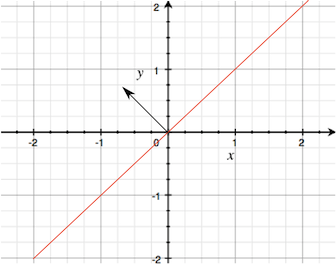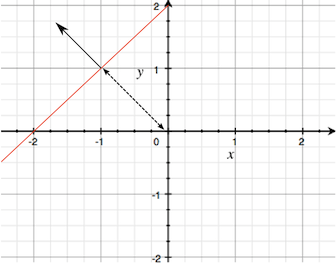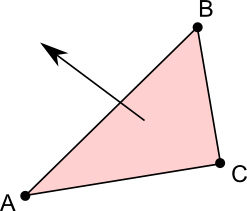Version: 2017.4
언어: 한국어

# Plane

struct in UnityEngine

매뉴얼로 전환

## 설명

Representation of a plane in 3D space.

A plane is an infinitely large, flat surface that exists in 3D space and divides the space into two halves known as half-spaces. It is easy to determine which of the two half-spaces a particular point is in and also how far the point is from the plane. Walls, floors and other flat surfaces are common in games, so a plane is sometimes useful for mathematical calculations with these objects. Also, there are cases where a real surface does not exist but it is useful to imagine that one is there. For example, in sports, a goal line or out-of-bounds line is often assumed to extend into the air, effectively defining a plane.

When a plane passes through the <0,0,0> point in world space, it is defined simply by a normal vector that determines which way it faces. It is easy to visualise this if you imagine looking at the plane edge-on.Note that the side from which the normal vector points is important since it is used to identify which half-space a point is in (ie, on the positive or "normal" side of the plane or the other side). When the plane doesn't pass through <0,0,0> it can be defined by the normal vector along with a distance from <0,0,0>A plane can also be defined by the three corner points of a triangle that lies within the plane. In this case, the normal vector points toward you if the corner points go around clockwise as you look at the triangle face-on..

## 변수

 distance Distance from the origin to the plane. flipped Returns a copy of the plane that faces in the opposite direction. normal Normal vector of the plane.

## 생성자

 Plane Creates a plane.

## Public 함수

 ClosestPointOnPlane For a given point returns the closest point on the plane. Flip Makes the plane face in the opposite direction. GetDistanceToPoint Returns a signed distance from plane to point. GetSide Is a point on the positive side of the plane? Raycast Intersects a ray with the plane. SameSide Are two points on the same side of the plane? Set3Points Sets a plane using three points that lie within it. The points go around clockwise as you look down on the top surface of the plane. SetNormalAndPosition Sets a plane using a point that lies within it along with a normal to orient it. Translate Returns a copy of the given plane that is moved in space by the given translation.